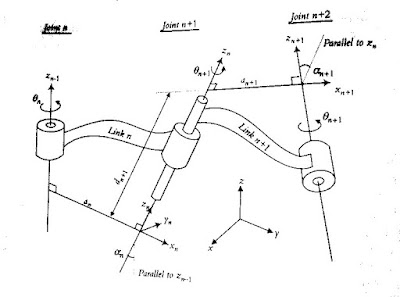## Tuesday, February 22, 2011

### Denavit-Hartenberg Representation of Robots

The D-H model of representation is a simple way of modeling robot links and joints that can be used for any robot configuration. We will have to assign a z-axis and an x-axis for each link. The D-H representation does not use the y-axis at all. Let Lk be the frame associated with link k.### 1. Define Z Axis from All Joints

From the axis of joint k+1, define the zk axis for link k. If the joint is revolute, the z axis is in the direction of rotation as followed by the right hand rule for the rotations. If the joint is prismatic, the z-axis for the joint is along the direction of the linear movement.

### 2. Define Origins

The intersection of the zk and zk-1 axes is selected as the origin of Lk . If they do not intersect, use the intersection of zk with a common normal between zk and zk-1. There is always one line mutually perpendicular to any two skew lines, called common normal, which is the shortest distance between them.

### 3. Define X-axis

Assign xk in the direction of the common normal between zk and zk-1. If z-axes are intersecting, select xk to be orthogonal to both zk and zk-1 (the direction of the cross-product of the two z-axes). If zk and zk-1 are parallel, point xk away from zk-1 colinear with the common normal of the previous joint.

### 4. Define Y-axis

Select yk to form a right-handed orthonormal coordinate frame Lk.

### 5. Define the Four Kinematic Parameters

k is defined as the angle between xk-1 and xk axes about the zk-1 axis. *dk is the distance between xk-1 and xk axes along zk-1 axis. *ak is the distance between zk-1 and zk axes along xk axis. *αk is the angle between zk-1 and zk axes about xk axis.

### 6. Transferring Frame k-1 to Frame k

*Rotation of Lk-1 about the zk-1 axis by θk will make xk-1 and xk parallel to each other. This is true because the common normals ak-1 and ak are both perpendicular to zk-1 axis. *Translation of Lk-1 along zk-1 axis a distance of dk will make xk-1 and xk colinear. *Translation of Lk-1 along xk axis a distance of ak will bring the origins of Lk-1 and Lk together. *Rotation of Lk-1 about xk axis by αk will make zk-1 and zk axes parallel. At this point, frames Lk-1 and Lk will be exactly the same.For simulation, a nice free software called Robotassist can be downloaded at www.kinematics.com

Ref:
Introduction to Robotics -Analysis, Control, Applications; Second Edition, Saeed Benjamin Niku
Fundamental of Robotics -Analysis & Control, Robert J. Schilling